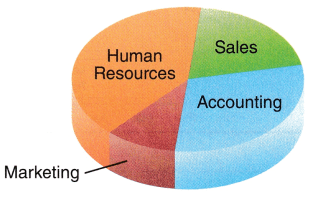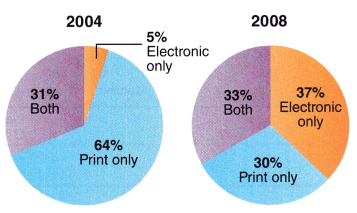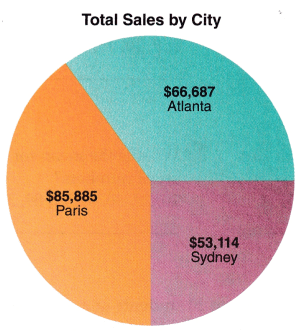# McGraw Hill Math Grade 6 Lesson 24.4 Answer Key Circle Graphs

Practice questions available in McGraw Hill Math Grade 6 Answer Key PDF Lesson 24.4 Circle Graphs will engage students and is a great way of informal assessment.

Exercises

INTERPRET

Question 1.
In the circle graph, which is the smallest department in terms of new hires for the year?Answer: From the given circle graph we observe that Marketing is the smallest department in terms of new hires for the year.

Question 2.
The circle graphs display how people in a company communicated in 2004 and 2008. What information can you gather from the two graphs?From the two graphs, we observed that Electronic has increased from 5% to 37% from 2004 to 2008.

Question 3.
Is the total of items A, E, and B more than C and D?A = 33%
B = 25%
C = 18%
D = 15%
E = 9%
A + E + B = 33 + 9 + 25 =67%
C + D = 18 + 15 = 33%
So, yes the total of items A, E, and B is more than C and D.

Question 4.
Do the Americas represent more than 50% of the total?No Americans do not represent more than 50% of the total.

Question 5.
According to the circle graph, are the combined sales for Atlanta and Sydney more than sales for Paris?Sales for Atlanta = $66,687 Sales for Paris =$85,885
Sales for Sydney = $53,114 66687 + 53,114 =$119801
So, the combined sales for Atlanta and Sydney are more than the sales for Paris.

Question 6.
In the circle graph, which age group makes up the majority of students who attend Basic Skills Summer School?